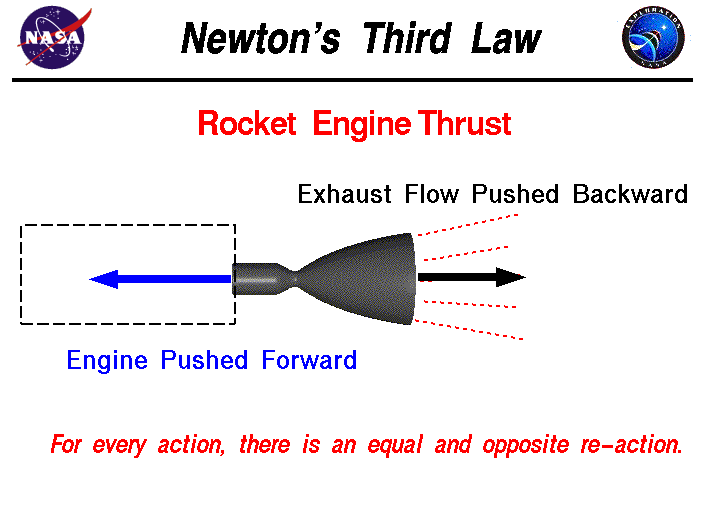Newton's Laws

Newton's 1st Law- Law of Inertia

- When no external forces act on a body (net force=0) the body will either remain at rest (v=0 ms-1) or continue to move with constant speed.Newton's first law is supported by the second law which states;

Newton's 2nd Law

- Force is equal to the product of mass and acceleration \$Fnet= ma\$

- hence when the change in velocity is equal to zero, the object will either remain at a constant speed or remain at rest. This can be seen from \$F=m ΔV/ Δt\$ because acceleration is equal to \$ΔV/ Δt\$Newton's 3rd Law

- If body A, exerts a force on body B, then body B will exert and equal but opposite force on body A.
These two resultant forces will have an equal magnitude but and opposite direction and the force will be exerted on different body's.Note: The action reaction pairs of forces do not cancel each other out because they are not applied in the same body.

By Rahill Ismael